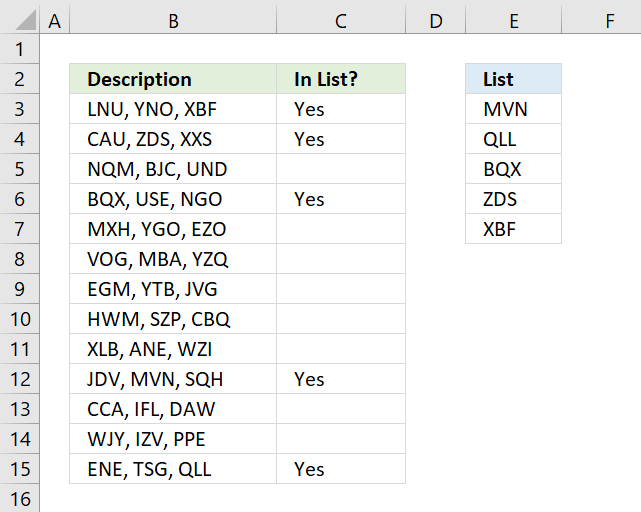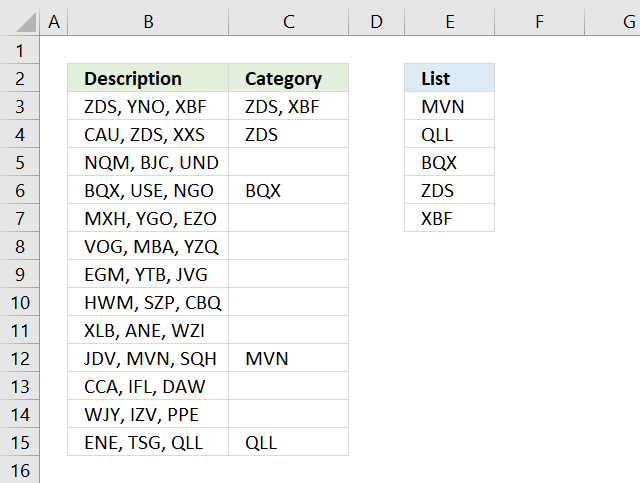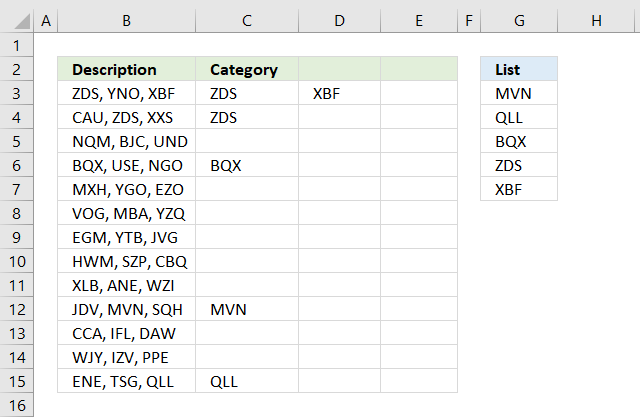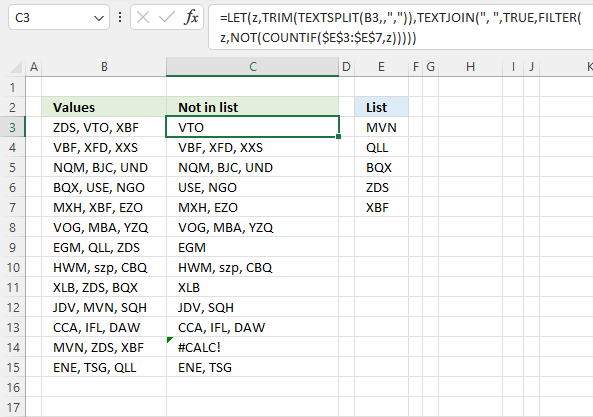Author: Oscar Cronquist Article last updated on February 01, 2023This article demonstrates several ways to check if a cell contains any value based on a list. The first example shows how to check if any of the values in the list is in the cell.

The remaining examples show formulas that also return the matching values. You may need different formulas based on the Excel version you are using.

Read this article If cell equals value from list to match the entire cell to any value from a list. To match a single cell to a single value read this: If cell contains text

To check if a cell contains all values in the list read this: If cell contains multiple values

## 1. Check if the cell contains any value in the listThe image above shows an array formula in cell C3 that checks if cell B3 contains at least one of the values in List (E3:E7), it returns "Yes" if any of the values are found in column B and returns nothing if the cell contains none of the values.

For example, cell B3 contains XBF which is found in cell E7. Cell B4 contains text ZDS found in cell E6. Cell C5 contains no values in the list.

=IF(OR(COUNTIF(B3,"*"&\$E\$3:\$E\$7&"*")), "Yes", "")

You need to enter this formula as an array formula if you are not an Excel 365 subscriber. There is another formula below that doesn't need to be entered as an array formula, however, it is slightly larger and more complicated.

1. Type formula in cell C3.
2. Press and hold CTRL + SHIFT simultaneously.
3.  Press Enter once.
4. Release all keys.

Excel adds curly brackets to the formula automatically if you successfully entered the array formula. Don't enter the curly brackets yourself.

### 1.1 Explaining formula in cell C3

#### Step 1 - Check if the cell contains any of the values in the list

The COUNTIF function lets you count cells based on a condition, however, it also allows you to count cells based on multiple conditions if you use a cell range instead of a cell.

COUNTIF(rangecriteria)

The criteria argument utilizes a beginning and ending asterisk in order to match a text string and not the entire cell value, asterisks are one of two wildcard characters that you are allowed to use.

The ampersands concatenate the asterisks to cell range E3:E7.

COUNTIF(B3,"*"&\$E\$3:\$E\$7&"*")

becomes

COUNTIF("LNU, YNO, XBF", {"*MVN*"; "*QLL*"; "*BQX*"; "*ZDS*"; "*XBF*"})

and returns this array

{0; 0; 0; 0; 1}

which tells us that the last value in the list is found in cell B3.

#### Step 2 - Return TRUE if at least one value is 1

The OR function returns TRUE if at least one of the values in the array is TRUE, the numerical equivalent to TRUE is 1.

OR({0; 0; 0; 0; 1})

returns TRUE.

#### Step 3 - Return Yes or nothing

The IF function then returns "Yes" if the logical test evaluates to TRUE and nothing if the logical test returns FALSE.

IF(TRUE, "Yes", "")

returns "Yes" in cell B3.

### Regular formula

The following formula is quite similar to the formula above except that it is a regular formula and it has an additional INDEX function.

=IF(OR(INDEX(COUNTIF(B3,"*"&\$E\$3:\$E\$7&"*"),)), "Yes", "")

### Get the Excel fileIF-cell-contains-text-from-list.xlsx

## 2. Display matches if the cell contains text from a listThe image above demonstrates a formula that checks if a cell contains a value in the list and then returns that value. If multiple values match then all matching values in the list are displayed.

For example, cell B3 contains "ZDS, YNO, XBF" and cell range E3:E7 has two values that match, "ZDS" and "XBF".

Formula in cell C3:

=TEXTJOIN(", ", TRUE, IF(COUNTIF(B3, "*"&\$E\$3:\$E\$7&"*"), \$E\$3:\$E\$7, ""))

The TEXTJOIN function is available for Office 2019 and Office 365 subscribers. You will get a #NAME error if your Excel version is missing this function. Office 2019 users may need to enter this formula as an array formula.

The next formula works with most Excel versions.

Array formula in cell C3:

=INDEX(\$E\$3:\$E\$7, MATCH(1, COUNTIF(B3, "*"&\$E\$3:\$E\$7&"*"), 0))

However, it only returns the first match. There is another formula below that returns all matching values, check it out.

How to enter an array formula

### 2.1 Explaining formula in cell C3

#### Step 1 - Count cells containing text strings

The COUNTIF function lets you count cells based on a condition, we are going to use multiple conditions. I am going to use asterisks to make the COUNTIF function check for a partial match.

The asterisk is one of two wild card characters that you can use, it matches 0 (zero) to any number of any characters.

COUNTIF(B3, "*"&\$E\$3:\$E\$7&"*")

becomes

COUNTIF("ZDS, YNO, XBF", {"*MVN*"; "*QLL*"; "*BQX*"; "*ZDS*"; "*XBF*"})

and returns {1; 0; 0; 0; 1}.

This array contains as many values as there values in the list, the position of each value in the array matches the position of the value in the list. This means that we can tell from the array that the first value and the last value is found in cell B3.

#### Step 2 - Return the actual value

The IF function returns one value if the logical test is TRUE and another value if the logical test is FALSE.

IF(logical_test, [value_if_true], [value_if_false])

This allows us to create an array containing values that exists in cell B3.

IF(COUNTIF(B3, "*"&\$E\$3:\$E\$7&"*"), \$E\$3:\$E\$7, "")

becomes

IF(COUNTIF("ZDS, YNO, XBF", {"*MVN*"; "*QLL*"; "*BQX*"; "*ZDS*"; "*XBF*"}), {"*MVN*"; "*QLL*"; "*BQX*"; "*ZDS*"; "*XBF*"}, "")

and returns {"";"";"";"ZDS";"XBF"}.

#### Step 3 - Concatenate values in array

The TEXTJOIN function allows you to combine text strings from multiple cell ranges and also use delimiting characters if you want.

TEXTJOIN(delimiterignore_emptytext1[text2], ...)

TEXTJOIN(", ", TRUE, IF(COUNTIF(B3, "*"&\$E\$3:\$E\$7&"*"), \$E\$3:\$E\$7, ""))

becomes

TEXTJOIN(", ", TRUE, {"";"";"";"ZDS";"XBF"})

and returns text strings ZDS, XBF.

## 3. Display matches if cell contains text from a list (Earlier Excel versions)The image above demonstrates a formula that returns multiple matches if the cell contains values from a list. This array formula works with most Excel versions.

Array formula in cell C3:

=IFERROR(INDEX(\$G\$3:\$G\$7, SMALL(IF(COUNTIF(\$B3, "*"&\$G\$3:\$G\$7&"*"), MATCH(ROW(\$G\$3:\$G\$7), ROW(\$G\$3:\$G\$7)), ""), COLUMNS(\$A\$1:A1))), "")

How to enter an array formula

Copy cell C3 and paste to cell range C3:E15.

### 3.1 Explaining formula in cell C3

#### Step 1 - Identify matching values in cell

The COUNTIF function lets you count cells based on a condition, we are going to use a cell range instead. This will return an array of values.

COUNTIF(\$B3, "*"&\$G\$3:\$G\$7&"*")

becomes

COUNTIF("ZDS, YNO, XBF", {"*MVN*"; "*QLL*"; "*BQX*"; "*ZDS*"; "*XBF*"})

and returns {0; 0; 0; 1; 1}.

#### Step 2 - Calculate relative positions of matching values

The IF function returns one value if the logical test is TRUE and another value if the logical test is FALSE.

IF(logical_test, [value_if_true], [value_if_false])

This allows us to create an array containing values representing row numbers.

IF(COUNTIF(\$B3, "*"&\$G\$3:\$G\$7&"*"), MATCH(ROW(\$G\$3:\$G\$7), ROW(\$G\$3:\$G\$7)), "")

becomes

IF({0; 0; 0; 2; 1}, MATCH(ROW(\$G\$3:\$G\$7), ROW(\$G\$3:\$G\$7)), "")

becomes

IF({0; 0; 0; 2; 1}, {1; 2; 3; 4; 5}, "")

and returns {""; ""; ""; 4; 5}.

#### Step 3 - Extract the k-th smallest number

I am going to use the SMALL function to be able to extract one value in each cell in the next step.

SMALL(arrayk)

SMALL(IF(COUNTIF(\$B3, "*"&\$G\$3:\$G\$7&"*"), MATCH(ROW(\$G\$3:\$G\$7), ROW(\$G\$3:\$G\$7)), ""), COLUMNS(\$A\$1:A1)))

becomes

SMALL({""; ""; ""; 4; 5}, COLUMNS(\$A\$1:A1)))

The COLUMNS function calculates the number of columns in a cell range, however, the cell reference in our formula grows when you copy the cell and paste to adjacent cells to the right.

SMALL({0; 0; 0; 4; 5}, COLUMNS(\$A\$1:A1)))

becomes

SMALL({""; ""; ""; 4; 5}, 1)

and returns 4.

#### Step 4 - Return value based on row number

The INDEX function returns a value from a cell range or array, you specify which value based on a row and column number. Both the [row_num] and [column_num] are optional.

INDEX(array[row_num][column_num])

INDEX(\$G\$3:\$G\$7, SMALL(IF(COUNTIF(\$B3, "*"&\$G\$3:\$G\$7&"*"), MATCH(ROW(\$G\$3:\$G\$7), ROW(\$G\$3:\$G\$7)), ""), COLUMNS(\$A\$1:A1)))

becomes

INDEX(\$G\$3:\$G\$7, 4)

becomes

INDEX({"MVN";"QLL";"BQX";"ZDS";"XBF"}, 4)

and returns "ZDS" in cell C3.

#### Step 5 - Remove error values

The IFERROR function lets you catch most errors in Excel formulas except #SPILL! errors. Be careful when using the IFERROR function, it may make it much harder spotting formula errors.

IFERROR(valuevalue_if_error)

There are two arguments in the IFERROR function. The value argument is returned if it is not evaluating to an error. The value_if_error argument is returned if the value argument returns an error.

IFERROR(INDEX(\$G\$3:\$G\$7, SMALL(IF(COUNTIF(\$B3, "*"&\$G\$3:\$G\$7&"*"), MATCH(ROW(\$G\$3:\$G\$7), ROW(\$G\$3:\$G\$7)), ""), COLUMNS(\$A\$1:A1))), "")

## 4. Filter delimited values not in the list (Excel 365)The formula in cell C3 lists values in cell B3 that are not in the List specified in cell range E3:E7. The formula returns #CALC! error if all values are in the list, see cell C14 as an example.

Excel 365 dynamic array formula in cell C3:

=LET(z,TRIM(TEXTSPLIT(B3,,",")),TEXTJOIN(", ",TRUE,FILTER(z,NOT(COUNTIF(\$E\$3:\$E\$7,z)))))

### 4.1 Explaining formula

#### Step 1 - Split values with a delimiting character

The TEXTSPLIT function splits a string into an array based on delimiting values.

Function syntax: TEXTSPLIT(Input_Text, col_delimiter, [row_delimiter], [Ignore_Empty])

TEXTSPLIT(B3,,",")

becomes

TEXTSPLIT("ZDS, VTO, XBF",,",")

and returns

{"ZDS"; " VTO"; " XBF"}

#### Step 2 - Remove leading and trailing spaces

The TRIM function deletes all blanks or space characters except single blanks between words in a cell value.

Function syntax: TRIM(text)

TRIM(TEXTSPLIT(B3,,","))

becomes

TRIM({"ZDS"; " VTO"; " XBF"})

and returns

{"ZDS"; "VTO"; "XBF"}

#### Step 3 - Check if values are in list

The COUNTIF function calculates the number of cells that is equal to a condition.

Function syntax: COUNTIF(range, criteria)

COUNTIF(\$E\$3:\$E\$7,TRIM(TEXTSPLIT(B3,,",")))

becomes

COUNTIF({"MVN";"QLL";"BQX";"ZDS";"XBF"},{"ZDS"; "VTO"; "XBF"})

and returns

{1; 0; 1}

These numbers indicate if a value is found in the list, zero means not in the list and 1 or higher means that the value is in the list at least once.

The number's position corresponds to the position of the values. {1; 0; 1} - {"ZDS"; "VTO"; "XBF"} meaning "ZDS" and "XBF" are in the list and "VTO" not.

#### Step 4 - Not

The NOT function returns the boolean opposite to the given argument.

Function syntax: NOT(logical)

NOT(COUNTIF(\$E\$3:\$E\$7,TRIM(TEXTSPLIT(B3,,","))))

becomes

NOT({1; 0; 1})

and returns

{FALSE; TRUE; FALSE}.

0 (zero) is equivalent to FALSE. The boolean opposite is TRUE.

Any other number than 0 (zero) is equivalent to TRUE.  The boolean opposite is FALSE.

#### Step 5 - Filter

The FILTER function extracts values/rows based on a condition or criteria.

Function syntax: FILTER(array, include, [if_empty])

FILTER(TRIM(TEXTSPLIT(B3,,",")),NOT(COUNTIF(\$E\$3:\$E\$7,TRIM(TEXTSPLIT(B3,,",")))))

becomes

FILTER({"ZDS"; "VTO"; "XBF"},{FALSE; TRUE; FALSE})

and returns

"VTO".

#### Step 6 - Join

The TEXTJOIN function combines text strings from multiple cell ranges.

Function syntax: TEXTJOIN(delimiter, ignore_empty, text1, [text2], ...)

TEXTJOIN(", ",TRUE,FILTER(TRIM(TEXTSPLIT(B3,,",")),NOT(COUNTIF(\$E\$3:\$E\$7,TRIM(TEXTSPLIT(B3,,","))))))

becomes

TEXTJOIN(", ",TRUE,{"VTO"})

and returns

"VTO".

#### Step 7 - Shorten the formula

The LET function lets you name intermediate calculation results which can shorten formulas considerably and improve performance.

Function syntax: LET(name1, name_value1, calculation_or_name2, [name_value2, calculation_or_name3...])

TEXTJOIN(", ",TRUE,FILTER(TRIM(TEXTSPLIT(B3,,",")),NOT(COUNTIF(\$E\$3:\$E\$7,TRIM(TEXTSPLIT(B3,,","))))))

z - TRIM(TEXTSPLIT(B3,,","))

LET(z,TRIM(TEXTSPLIT(B3,,",")),TEXTJOIN(", ",TRUE,FILTER(z,NOT(COUNTIF(\$E\$3:\$E\$7,z)))))